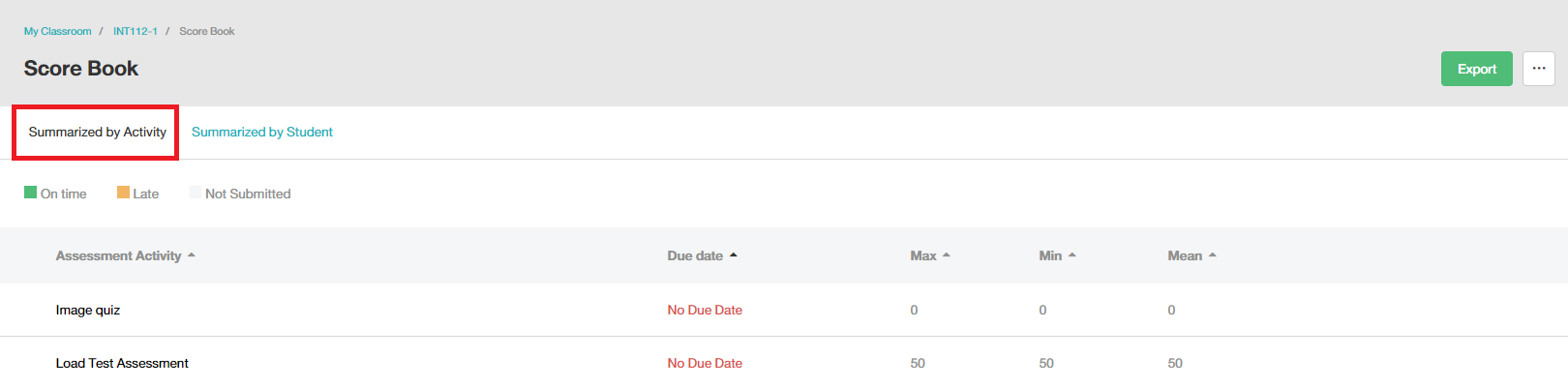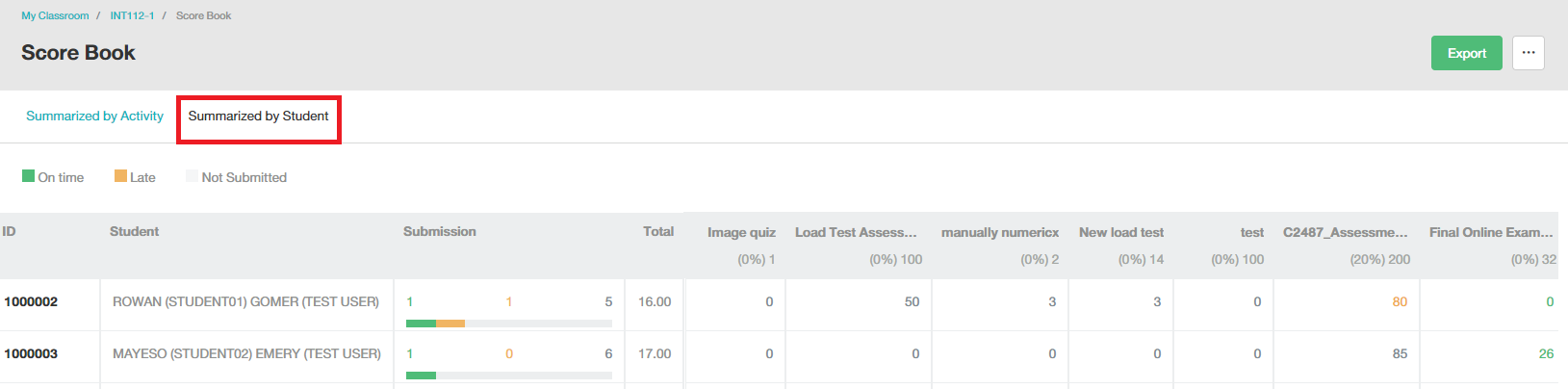# How is the score shown in Scorebook

• Updated

Q: How is the score shown in Score book?

A: In feature Score book, the score is shown as summarized by activity and summarized by student.

1. Summarized by activity
• Show max, min and mean score of the class
• Show the submission status of the class
• Be able to export score as an excel file2.  Summarized by student

• Show the score of each student
• Show the total score of all activities of each student
• Show the score in each activity
• Show submission status of each student
• Be able to export score as an excel fileRelated Article >> Summarized score